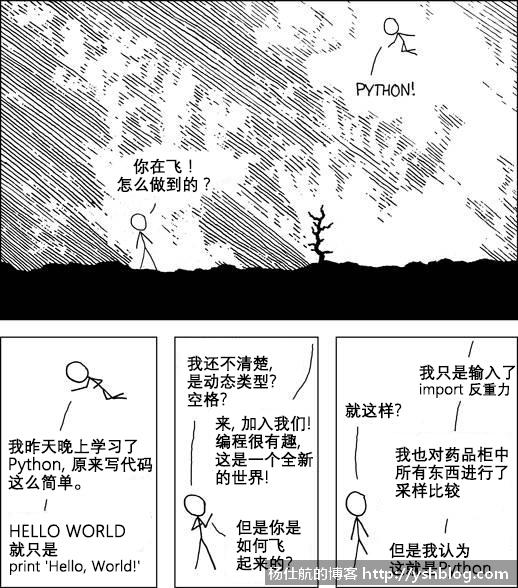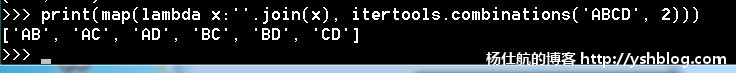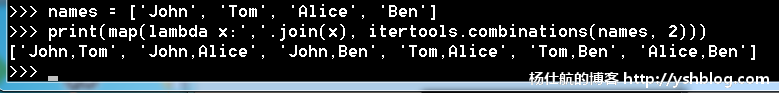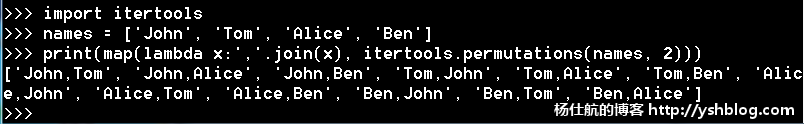### Python轻松实现排列和组合

• 发布时间：2016年12月26日 10:07
• 作者：杨仕航
• 分类标签： Python
• 阅读(24046)
• 评论(0)```#coding:utf-8
import itertools

#使用permutations方法
ls = itertools.combinations('ABCD', 2)

#遍历所有组合情况
for l in ls:
print(l)```combinations方法第1个参数是需要组合的数据，第2个参数是组合的个数。

```#coding:utf-8
import itertools

print(map(lambda x:''.join(x), itertools.combinations('ABCD', 2)))``````#coding:utf-8
import itertools

names = ['John', 'Tom', 'Alice', 'Ben']
print(map(lambda x:','.join(x), itertools.combinations(names, 2)))``````#coding:utf-8
import itertools

names = ['John', 'Tom', 'Alice', 'Ben']
print(map(lambda x:','.join(x), itertools.permutations(names, 2)))```itertools是一个实用的模块，里面还有很多方法没有提及。大家有兴趣可以自行了解。